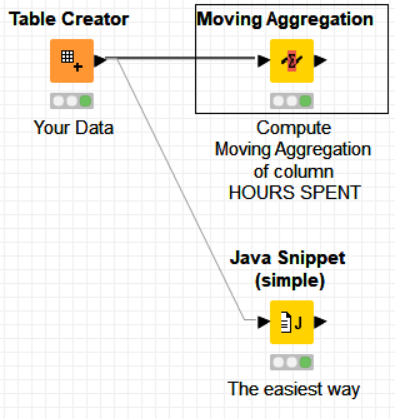# How to loop through rows and use a calculation of the last row

Hi all,

I have the following situation that I am strugling with.

I have a data set like this:

|| HOURS SPENT || TOTAL TIME ||
|| 0.1 || 0.1 ||
|| 0.2 || ? ||
|| 0.3 || 0.6 ||
|| 0.1 || ? ||
|| 0.2 || ? ||
|| 0.3 || ? ||
|| 0.1 || 1.3 ||

My goal is to go through each row and apply the folowing logic:

If “TOTAL TIME” is not null then “TOTAL TIME” = “TOTAL TIME”
If “TOTAL TIME” is null then “TOTAL TIME” = “TOTAL TIME” (previous row) + “HOURS SPENT”

I know I can use the missing value node to get the previous “TOTAL TIME” (previous row), the issue is when I have multiple rows where “TOTAL TIME” is null, then the missing value node will copy the last known value, which is not what I need.

So basically I would need to make the if logic in the first row, then update the “TOTAL TIME” of that row, then use that value for the next row logic, and so on.

In the end I want something like this:

|| HOURS SPENT || TOTAL TIME ||
|| 0.1 || 0.1 ||
|| 0.2 || 0.3 ||
|| 0.3 || 0.6 ||
|| 0.1 || 0.7 ||
|| 0.2 || 0.9 ||
|| 0.3 || 1.2 ||
|| 0.1 || 1.3 ||

I think this may be able to be achieve through loops but I am strugling to find the right way to solve this problem.

Any help will be appreciated!

I believe one does not need here column “TOTAL TIME” to calculate its values. Just with column “HOURS SPENT” one can get the values of “TOTAL TIME”.

The operation needed here is a moving aggregation with “SUM” as aggregation function:

The workflow is as follows:

Hope it helps.

Best
Ael

1 Like

Hi Ael,

Thanks for the help. Indeed that is a way to go, but in my case the “TOTAL TIME” column not always correspond with the moving agregate sum of “TIME SPENT” from the begining.
That is indeed one of the checks that I need to perform on the data set afterwards.
That is why I need to use the logic as described in my initial post.

Any way to achieve that?

Thanks,
Bruno

Maybe this other solution does the trick:Hope it helps.

Best
Ael

5 Likes

To get the previous value you could also apply LAG column node and build your logic from there
br

Hi Aworker.

This did the trick. Thanks for the help!

1 Like

If you think the solution is worthwhile, please check as solution the last message with the workflow so that other people can find it easily when having the same problem